## Get Address of First Cell in Range

We will learn how to get address of first cell in range in this post. At Instance, we must determine the address of first cell in range to use as the data table’s value. For the DATA table, we have a range of value values in the form of an array that is sent to the formula as input, and therefore the formula returns the first cell value.

The next sections include information on the functions mentioned before that we will use.

ADDRESS retrieves the address of a cell using the row and column numbers.

## Syntax:

`=ADDRESS(row_num, col_num, [abs_num] )`
• `Row_num`: The Row number
• `Col_num`: The Column number
• `[abs num]`: [Optional] integer, one of the following values: 1, 2, 3, or 4. If this parameter is ignored or set to 1, the returned address will be absolute, e.g. \$A\$1.

Now we’ll combine these functions into a formula. We’ll begin with a data table. We need to determine the data table’s initial cell address.

Utilize the following formula:

`=ADDRESS(ROW(table_array), COLUMN(table_array))`

Variables:

• `table array`: array representation of a data table

## Examples:

Let us validate this formula by applying it to an example.

Here we have a data table and we need to use the formula to get the first cell address in the same data table.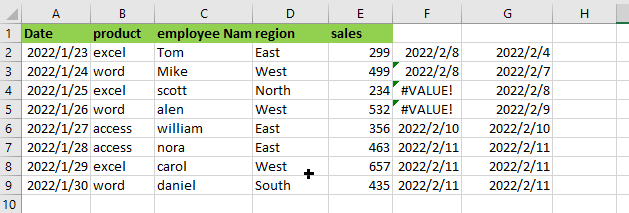Use the following Formula:

`= ADDRESS(ROW(A1:E9), COLUMN(A1:E9))`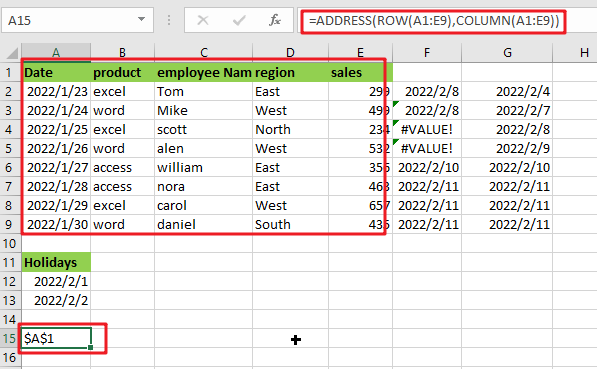Note: A1:E9 is array of tables

## Let’s See How This Formula Works:

`= COLUMN(A1:E9)`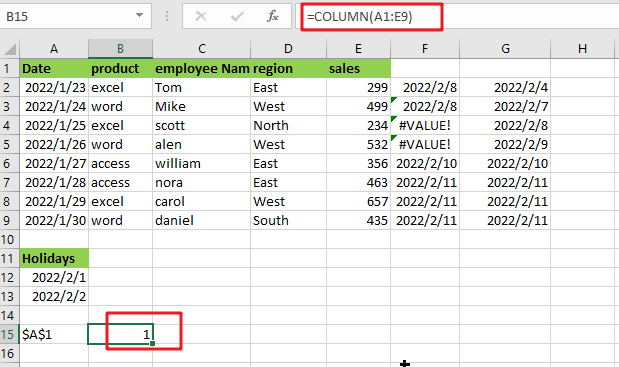The COLUMN function returns the value of the first column in the table array.

`= ROW(A1:E9)`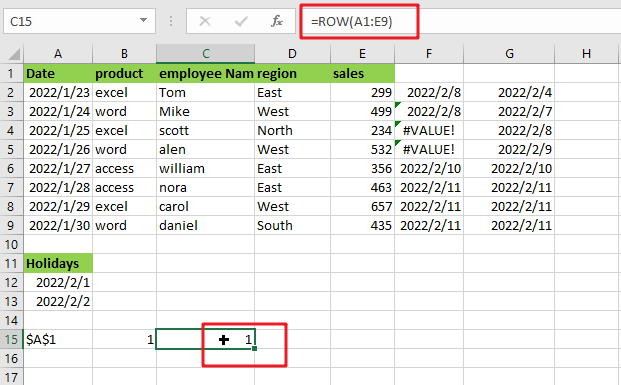The ROW function returns the index of the first row in the table array.

The ADDRESS function accepts the row number and column value parameters and returns the row’s and column’s absolute references.

The array sent to the function is denoted by cell reference. To get the result, press Enter.

As you can see, the formula works correctly since it delivers the value for the first cell in the data table.

Alternatively, you may use the CELL function:

CELL is an Excel built-in information function. Excel’s Cell function saves all the data in a specified cell and returns the cell’s info type. Using the follow formula:

`=CELL("address", [array_reference])`

or

`=CELL("address",INDEX(A1:E9,1,1))`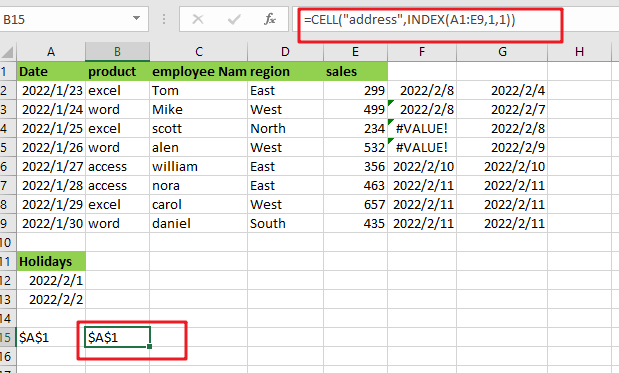Note:

• `[array_reference] `: table array given as reference.

As seen by the above calculation, you may use the first cell value as a reference.

The INDEX function can be used to get cell reference to the first cell in the given range by giving INDEX 1 for row number and 1 for column number.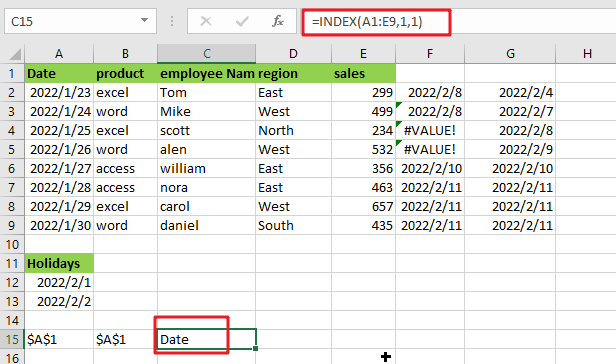The ADDRESS function may be used in conjunction with the ROW, COLUMN, and MIN functions to get the address of the first cell in a range. I hope this post on finding the get address of first cell in range in Excel was informative. It’s extremely easy and very helpful for people who use excel regularly.

### Related Functions

• Excel INDEX function
The Excel INDEX function returns a value from a table based on the index (row number and column number)The INDEX function is a build-in function in Microsoft Excel and it is categorized as a Lookup and Reference Function.The syntax of the INDEX function is as below:= INDEX (array, row_num,[column_num])…
• Excel ROW function
The Excel ROW function returns the row number of a cell reference.The ROW function is a build-in function in Microsoft Excel and it is categorized as a Lookup and Reference Function.The syntax of the ROW function is as below:= ROW ([reference])….
The Excel ADDRESS function returns a reference as a text string to a single cell.The syntax of the ADDRESS function is as below:=ADDRESS (row_num, column_num, [abs_num], [a1], [sheet_text])….
• Excel COLUMN function
The Excel COLUMN function returns the first column number of the given cell reference.The syntax of the COLUMN function is as below:=COLUMN ([reference])….
• Excel CELL function
The Excel CELL function returns information about the formatting, location, size, or contents of a cell.The syntax of the CELL function is as below:= CELL (info_type,[reference])…
• Excel MIN function
The Excel MIN function returns the smallest numeric value from the numbers that you provided. Or returns the smallest value in the array.The MIN function is a build-in function in Microsoft Excel and it is categorized as a Statistical Function.The syntax of the MIN function is as below:= MIN(num1,[num2,…numn])….

## How to Get the Active Cell Address in Excel

This post will guide you how to get the active Cell address with a formula in Excel. How do I return address of active cell with a VBA Macro in Excel.

## Get the Active Cell Address with Formula

If you want to return the address of an active cell in your current worksheet, you can use a formula based on the ADDRESS function, the ROW function and the COLUMN Function to achieve the result. The formula is shown as below:

`=ADDRESS(ROW(),COLUMN())`

You just need to select a cell and make it as active cell, then enter this formula into the formula bar, press Enter key on your keyboard. You would notice that the address of active cell is returned.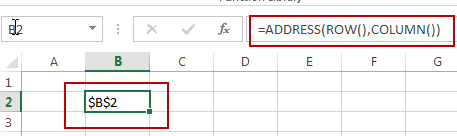## Get the Active Cell Address with VBA

You can also use an Excel VBA Macro to achieve the same result of returning the active cell address to a given cell. You just need to do the following steps:

#1 right click on the sheet tab in your worksheet, and select View Code from the popup menu list. And the Microsoft Visual Basic for Application window will appear. Or you can directly press Alt+ F11 to launch the VBA window.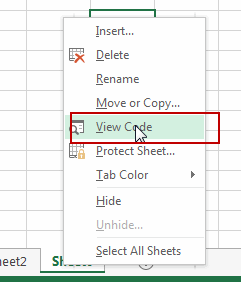#2 type the following code into the code window, then click Save button to save it.

```Private Sub Worksheet_SelectionChange(ByVal Target As Range)
End Sub```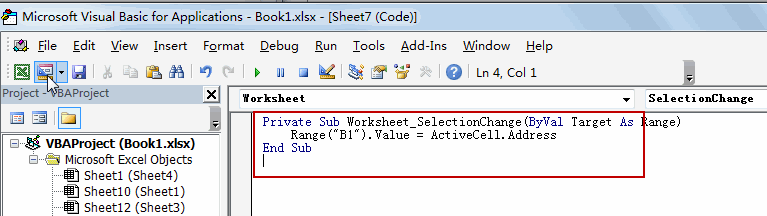#3 close VBA window and back to your worksheet. You can try to click or select one cell, the cell address of active cell is shown in Cell B2.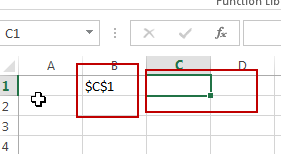### Related Functions

The Excel ADDRESS function returns a reference as a text string to a single cell.The syntax of the ADDRESS function is as below:=ADDRESS (row_num, column_num, [abs_num], [a1], [sheet_text])….
• Excel COLUMN function
The Excel COLUMN function returns the first column number of the given cell reference.The syntax of the COLUMN function is as below:=COLUMN ([reference])….
• Excel ROW function
The Excel ROW function returns the row number of a cell reference.The ROW function is a build-in function in Microsoft Excel and it is categorized as a Lookup and Reference Function.The syntax of the ROW function is as below:= ROW ([reference])….

## How to convert Column Letter to Number in Excel

This post will guide you how to convert column letter to number in Excel. How do I convert letter to number with a formula in Excel. How to convert column letter to number with VBA macro in Excel. How to use a User Defined Function to convert letter to number in Excel.

## Convert Column Letter to Number with a Formula

If you want to convert a column letter to a regular number, and you can use a formula based on the COLUMN function and the INDIRECT function to achieve the result.

For example, you need to convert a column letter in Cell B1 to number

Just like this:

`=COLUMN(INDIRECT(B1&1))`

Type this formula into a blank cell such as: C1, and press Enter key, and then drag the AutoFill Handle over to other cells to apply this formula.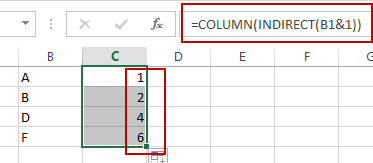The INDIRECT function will convert the text into a proper Excel reference and then pass the result to Column function to get the column number for the reference.

## Convert Column Letter to Number with VBA Macro

You can also use an Excel VBA Macro to achieve the result of converting column letter into its corresponding numeric value. Just do the following steps:

#1 open your excel workbook and then click on “Visual Basic” command under DEVELOPER Tab, or just press “ALT+F11” shortcut.#2 then the “Visual Basic Editor” window will appear.

#3 click “Insert” ->”Module” to create a new module.#4 paste the below VBA code into the code window. Then clicking “Save” button.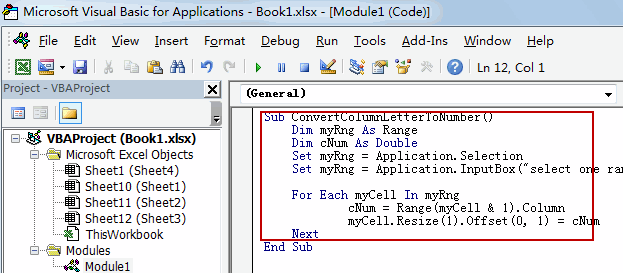```Sub ConvertColumnLetterToNumber()
Dim myRng As Range
Dim cNum As Double
Set myRng = Application.Selection
Set myRng = Application.InputBox("select one range that contain column letters", "ConvertColumnLetterToNumber", myRng.Address, Type:=8)

For Each myCell In myRng
cNum = Range(myCell & 1).Column
myCell.Resize(1).Offset(0, 1) = cNum
Next
End Sub```

#5 back to the current worksheet, then run the above excel macro. Click Run button.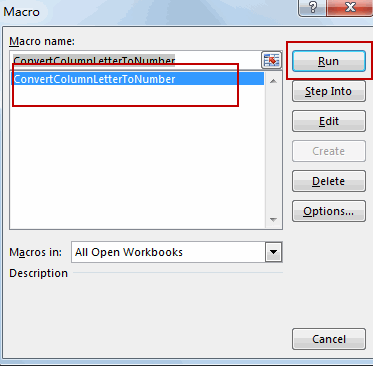#6 select one range that contain column letters, such as B1:B4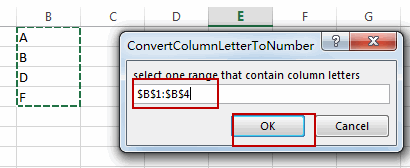#7 let’s see the result: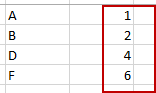## Convert Column Letter to Number with User Defined Function

Excel does not have a built in formula to convert column letter to a numeric number, but you can write down a User Defined Function to achieve the result. Just do the following steps:

#1 repeat the above step 1-3

#2 paste the below VBA code into the code window. Then clicking “Save” button.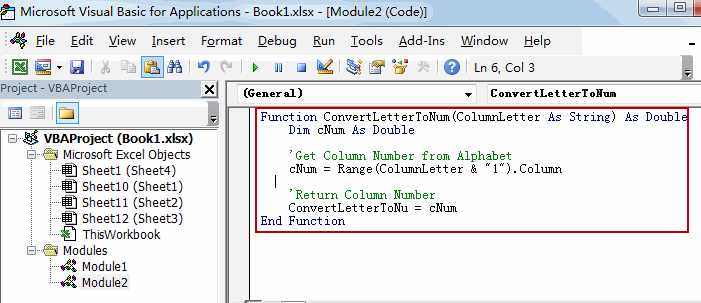```Function ConvertLetterToNum(ColumnLetter As String) As Double
Dim cNum As Double

'Get Column Number from Alphabet
cNum = Range(ColumnLetter & "1").Column

'Return Column Number
ConvertLetterToNum = cNum
End Function```

#3 back to the current worksheet, then type the following formula in a blank cell. press Enter key.

=ConvertLetterToNum(B1)

#4 select the cell C1, and  drag the AutoFill Handle over to other cells to apply this formula.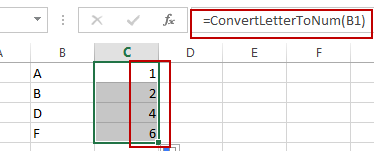## Convert Number to Column Letter

If you want to convert column number to an Excel Column letter, you can use another formula based on the SUBSTITUTE function and the ADDRESS function. Just like this:

`=SUBSTITUTE(ADDRESS(1,C1,4),"1","")`

Type this formula into Cell D1, and press Enter key. And then drag the AutoFill handle over to other cells to apply this formula.

Let’s see the last result: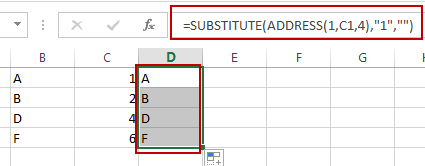Video: Convert Column Letter to Number

### Related Functions

• Excel Substitute function
The Excel SUBSTITUTE function replaces a new text string for an old text string in a text string.The syntax of the SUBSTITUTE function is as below:= SUBSTITUTE  (text, old_text, new_text,[instance_num])….
The Excel ADDRESS function returns a reference as a text string to a single cell.The syntax of the ADDRESS function is as below:=ADDRESS (row_num, column_num, [abs_num], [a1], [sheet_text])….
• Excel INDIRECT function
The Excel INDIRECT function returns the cell reference based on a text string, such as: type the text string “A2” in B1 cell, it just a text string, so you can use INDIRECT function to convert text string as cell reference….
• Excel COLUMN function
The Excel COLUMN function returns the first column number of the given cell reference.The syntax of the COLUMN function is as below:=COLUMN ([reference])….

## Get Cell Value Based on Row and Column Numbers

This post will guide you how to retrieve values using references based on Row and Column numbers in excel. How to get the cell value based on row and column numbers with an excel formula or user defined function in excel.

## Get Cell Value Based on Row and Column Numbers

If you want to retrieve values using cell references based on row and column numbers, you can create a formula based on the INDIRECT function and the ADDRESS function.

Assuming that there is a row number in cell D1 and there is a column number in cell D2, if you want to retrieve the cell value based on the specified row number and column number, just type the following formula in cell D3:

`=INDIRECT(ADDRESS(D1,D2))`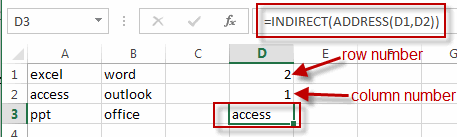You can also write a User Defined Function to get content of a cell given the row and column numbers. Just do the following steps:

#1 open your excel workbook and then click on “Visual Basic” command under DEVELOPER Tab, or just press “ALT+F11” shortcut.#2 then the “Visual Basic Editor” window will appear.

#3 click “Insert” ->”Module” to create a new module#4 paste the below VBA code into the code window. Then clicking “Save” button.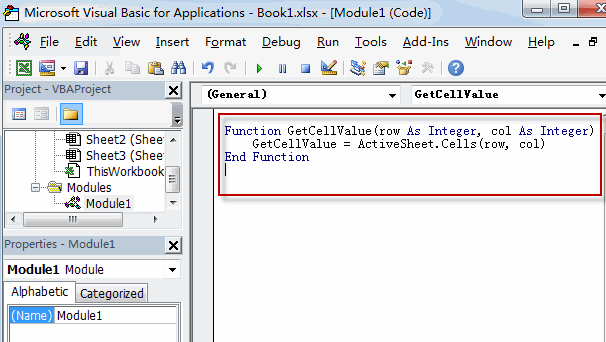```Function GetCellValue(row As Integer, col As Integer)
GetCellValue = ActiveSheet.Cells(row, col)
End Function```

#5 back to the current worksheet, try to enter the below formula in Cell D4.

`=GetCellValue(2,1)`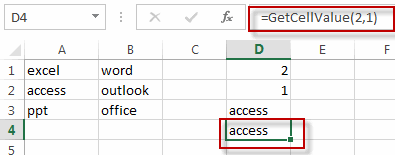### Related Functions

The Excel ADDRESS function returns a reference as a text string to a single cell.The syntax of the ADDRESS function is as below:=ADDRESS (row_num, column_num, [abs_num], [a1], [sheet_text])….
• Excel INDIRECT function
The Excel INDIRECT function returns the cell reference based on a text string, such as: type the text string “A2” in B1 cell, it just a text string, so you can use INDIRECT function to convert text string as cell reference….

## How to convert column number to letter

This post explains that how to convert a column number to a column letter using formula in excel. How to convert column number to a column letter using user defined function in VBA.

## Convert column number to letter using excel formula

if you want to convert column number to letter, you can use the ADDRESS function to get the absolute reference of one excel cell that contains that column number, such as, you can get the address of Cell B1, then you can use the SUBSTITUTE function to replace row number with empty string, so that you can get the column letter. So you can write down the following formula using the SUBSTITUTE function and the ADDRESS function.

`=SUBSTITUTE(ADDRESS(1,B1,4),"1"," ")`

Let’s see how this formula works: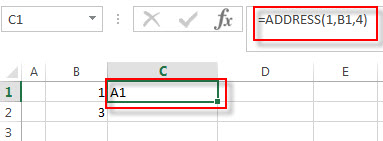The ADDRESS function returns an absolute reference for a cell at a given row and column number. And this formula returns A1. The returned address goes into the SUBSTITUTE function as its Text argument.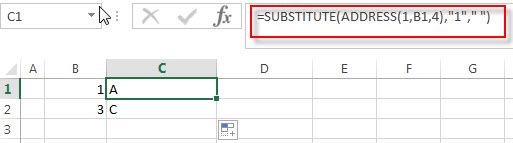This formula will replace the old_text “1” with empty string for a string of cell reference returned by the ADDRESS. So it returns a letter.

## Convert column number to letter with VBA user defind function

You can also create a new user defined function to convert column number to  a column letter in Excel VBA:

1# click on “Visual Basic” command under DEVELOPER Tab.2# then the “Visual Basic Editor” window will appear.

3# click “Insert” ->”Module” to create a new module named4# paste the below VBA code into the code window. Then clicking “Save” button.

```Public Function ConNumToLetter(Collet)
End Function```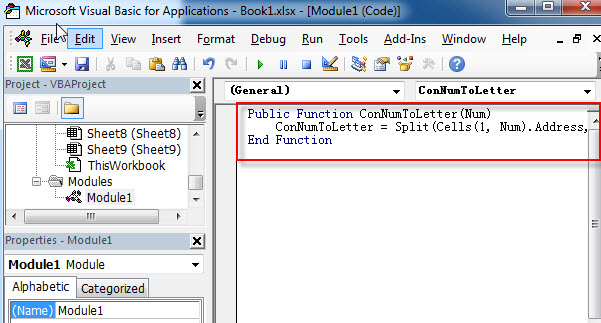5# back to the current worksheet, then enter the below formula in Cell C1:

`= ConNumToLetter(B1)`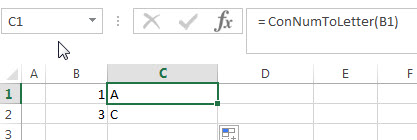### Related Formulas

• Extract text after first comma or space
If you want to get substring after the first comma character from a text string in Cell B1, then you can create a formula based on the MID function and FIND function or SEARCH function ….
• Convert column letter to number
If you want to convert a column letter to number, you can use a combination of the COLUMN function and the INDIRECT function to create an excel formula…..

### Related Functions

The Excel ADDRESS function returns a reference as a text string to a single cell.The syntax of the ADDRESS function is as below:=ADDRESS (row_num, column_num, [abs_num], [a1], [sheet_text])….
• Excel Substitute function
The Excel SUBSTITUTE function replaces a new text string for an old text string in a text string.The syntax of the SUBSTITUTE function is as below:= SUBSTITUTE  (text, old_text, new_text,[instance_num])….

This post will guide you how to use Excel ADDRESS function with syntax and examples in Microsoft excel.

### Description

The Excel ADDRESS function returns a reference as a text string to a single cell.

The ADDRESS function is a build-in function in Microsoft Excel and it is categorized as a Lookup and Reference Function.

The ADDRESS function is available in Excel 2016, Excel 2013, Excel 2010, Excel 2007, Excel 2003, Excel XP, Excel 2000, Excel 2011 for Mac.

### Syntax

The syntax of the ADDRESS function is as below:

`=ADDRESS (row_num, column_num, [abs_num], [a1], [sheet_text])`

Where the ADDRESS function arguments are:

• row_num -This is a required argument. The row number to use in the cell reference.
• column_num – This is a required argument. The column number to use in the cell reference.
• Abs_num – This is an optional argument. It will specify the type of reference to use. The following values can be used:

1 – Absolute

2 – Absolute row; relative column

3 – Relative row; absolute column

4 – Relative

A1 – This is an optional argument. It will specify the style of reference to use.

• True– A1 reference style
• False – R1C1 reference style

Sheet_text – This is a required argument. The sheet name to use.

### Example

The below examples will show you how to use Excel ADDRESS Lookup and Reference Function to return a reference as a text.

#1 Absolute reference, type formula: =ADDRESS(3,4)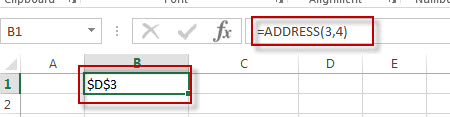#2 Absolute row, relative column, type formula: =ADDRESS(3,4,2)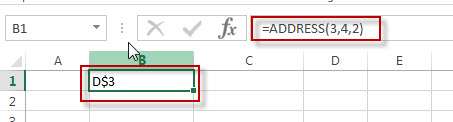#3 Absolute row, relative column in R1C1 reference style, type formula:=ADDRESS(3,4,2,FALSE)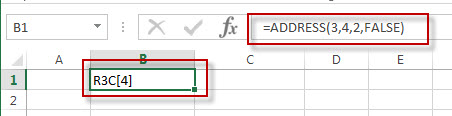## Excel Lookup And Reference Functions

This section will learn  how to use Excel’s Lookup And Reference Functions such as: Address, Areas, Columns, Hlookup, Vlookup, Lookup, Index, Match, etc.

ADDRESS – returns a reference as a text string to a single cell.

AREAS – returns the number of ranges in a reference.

CHOOSE – returns a value from a list of values.

COLUMN – returns the first column number of the given cell reference.

COLUMNS – returns the number of columns in an Array or a reference.

HLOOKUP – lookup a value in the top row of the table and return the value in the same column based on index_num position.

HYPERLINK – creates a shortcut/hyperlink to a document, when you click this hyperlink, the excel will open the file that is stored on a network server or local location.

INDEX – returns a value from a table based on the index (row number and column number)

INDIRECT – returns the cell reference based on a text string, such as: type the text string “A2” in B1 cell, it just a text string, so you can use INDIRECT function to convert text string as cell reference.

LOOKUP – search a value in a vector or array.

MATCH – search a value in an array and returns the position of that item.

ROW – returns the row number of a cell reference.

ROWS – returns the number of rows in a cell reference.

VLOOKUP – lookup a value in the first column of the table and return the value in the same row based on index_num position.